A convex spherical mirror has a radius of curvature of magnitude36.0cm. (a) Determine the position of the virtual image and the

Question

A convex spherical mirror has a radius of curvature of magnitude36.0cm.

(a) Determine the position of the virtual image and the magnification for object distances of25.0cm. Indicate the location of the image with the sign of your answer.
image location =cm
magnification =
(b) Determine the position of the virtual image and the magnification for object distances of47.0cm. Indicate the location of the image with the sign of your answer.
image location =cm
magnification =
(c) Are the images in parts (a) and (b) upright or inverted?
The image in part (a) is—Select—uprightinverted
The image in part (b) is—Select—uprightinverted

in progress 0
5 months 2021-08-06T10:07:52+00:00 1 Answers 0 views 0

Explanation:

a )

focal length of convex spherical mirror

f = 36/2  cm = 18 cm

object distance u = – 25 cm

mirror formula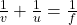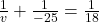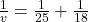v = 6.28  cm .

It is positive hence the image will be erect / upright  and formed on the back of the mirror.

For object distance of 47 cm

u = – 47 cm

Putting the values in the mirror formula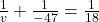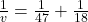v = 13  cm

It is positive hence the image will be erect / upright and formed on the back of the mirror.